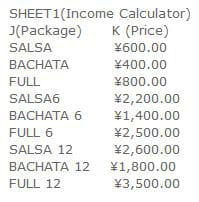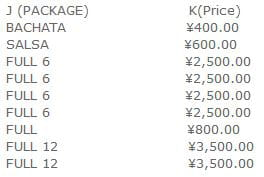# Students Package Payment control

Closed
Mau Mau - Nov 17, 2015 at 03:44 AM
Hello, I have the following columnsI enter the student information like name contact number etc,
I have a column J(Package) in which I set up a Validarion list with the values on
"Column A(Package)" from Sheet 1 so any time a new student sign up I can chose from the drop down list which package has he/she has paid I want column K(price) to show the right price based upon the package that has been input on column
J (Package) at any moment.I've tried the formula
=VLOOKUP(J5,'INCOME CALCULATOR'!J27:K35,2,FALSE)

but when I drag down the formula to the other cells in column K then
the values on the formula start adding up and then the formula stops being valid
cause it also changes the range of the selected array 'INCOME CALCULATOR'!J27:K35

example:
=VLOOKUP(J5,'INCOME CALCULATOR'!J27:K35,2,FALSE)
=VLOOKUP(J6,'INCOME CALCULATOR'!J28:K36,2,FALSE)
=VLOOKUP(J7,'INCOME CALCULATOR'!J29:K37,2,FALSE)
=VLOOKUP(J8,'INCOME CALCULATOR'!J30:K38,2,FALSE)... etc.

Thank you very much in advance any help is highly appreciated

Kind regards;